Next: Clifford Tori and Hopf Up: The Sphere in Three Previous: Visualizing the N-Sphere

Subsections

# Inversion

## Inverting
inside
From our two balls model, it is easy to see that we can shrink the equatorialinside, until it is a small sphere surrounding the north pole or the south pole.

Let
, and let
such that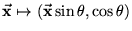. Then varying
in the range
gives a continuous family ofinside. When
or
, the sphere is shrunk to a single point. Whenis close to 1 or -1, it's a little sphere around either the north or south pole. Whenis near zero, it is nearly equatorial.

If we now consider the two regions into which theis divided by this moving, this might seem very similar to some process of turning theinside out.'' However, this is not quite correct. We might look at theinsideat some point and label the two regions as inside'' and outside'' based on which region was smaller. We do this because we are used to Euclidean space, where the inside,'' of a closed region usually appears smaller, and the outside usually includes the point at infinity.

However, the region inside the sphere is already finite, and so calling this process inverting,'' or swapping the inside'' and outside'' ofinsidereally doesn't make a whole lot of sense. All we can say is that we can distortinsidein such a way as to shrink one region down to a point while expanding another region from a point to the whole sphere.

Following from this, we see that any closed surface insidecan be inverted,'' by simply gluingto a small region of the manifold, and then inverting'' this sphere.

From now on I'll use the word inversion'' in the sphere to mean a process like this which apparently reverses the two regions increated by a dividing surface in.

## Inverting
insideNotice that
and
are identical manifolds. So we know, trivially, that we can invertinside
.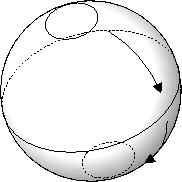Notice also that we can embed the flat torus,
, inside
. To see this best, consider our space to be
. Then the sphere consists of all points
such that
, and the torus consists of all pointssuch that
.

Inside, this torus can be inverted,'' that is, it can be deformed in such a way so that (1) the generators of the torus are swapped, and (2) the apparent outside'' is swapped with the apparent inside.'' Here are a series of pictures to illustrate the second point; pictures showing the generators being swapped are in section 4.2.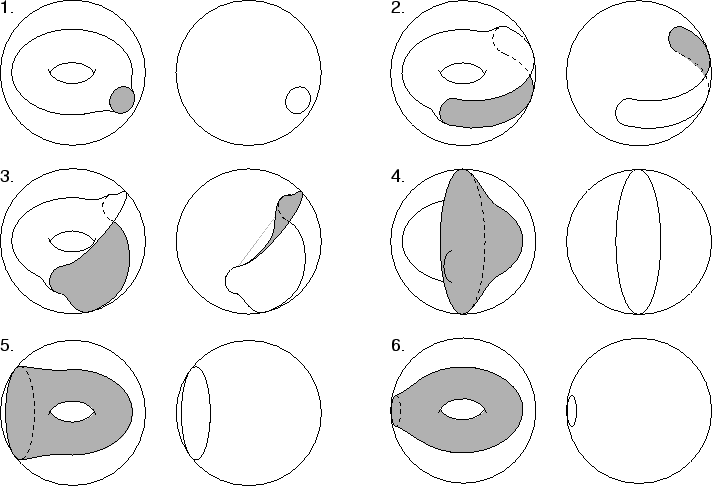The important point to notice from this is thatdividesinto two regions, both of which are topologically equivalent to a filled. It is by no means obvious that this is a general fact aboutinside. Furthermore, we can ask whether there is any deeper structure behind this inversion, or whether it is merely a manifestation of the fact, noted above, that any manifold can be inverted inside of the sphere.

### InvertinginsideWe know that any manifold can be inverted insideby gluing it to a sphere. If we know thatin general can be embedded inside, then we will know thatcan be inverted inside, by gluing the torus to aand inverting that.

The proof is inductive. To begin with, we know thatcan be embedded in
, which can be stereographically projected to.

If, in general,can be embedded in
, then we can embed
in. Think of this simply as as a thickened'' or fattened'' torus. Next, we cross both with
, to get
. Now, we can draw a little circle in
to see that
. And, of course,
. So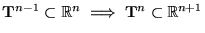. Since
can be embedded in
,can be embedded in
as we can just projecttovia stereographic projection.

So, in general,can be embedded and inverted'' inside. The next question is whether the neat symmetry ofingeneralizes as well. To do this we will need to look closer at the structure of.Next: Clifford Tori and Hopf Up: The Sphere in Three Previous: Visualizing the N-Sphere